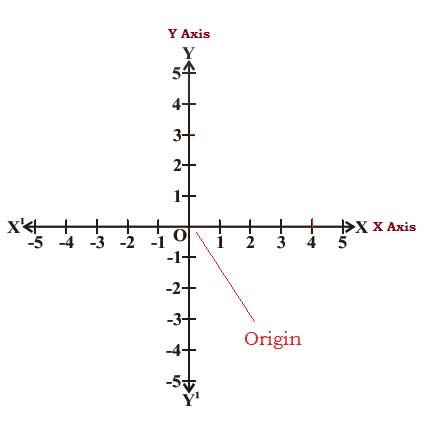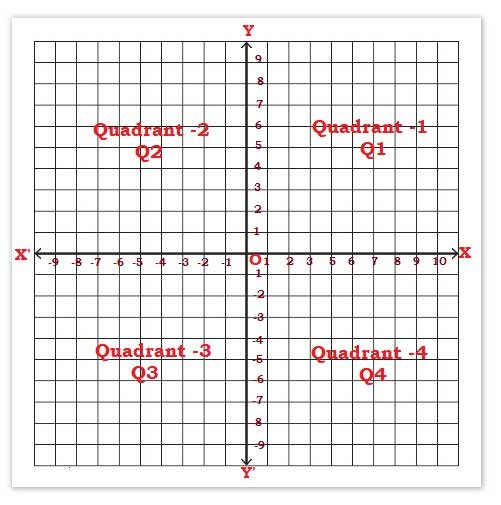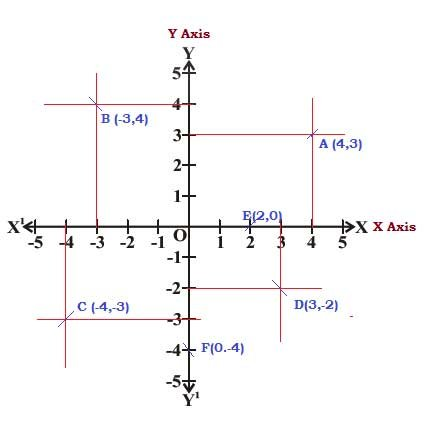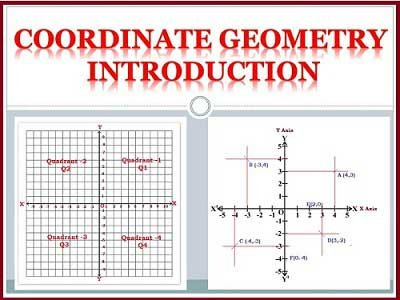# Coordinate geometry introduction | Locating of coordinate points

In this article we will get an idea about initial understanding of Coordinate geometry concepts like cartesian plane, quadrants, X-coordinate (abscissa), Y-coordinate (ordinate) & how to plot the points on a co-ordinate plane.

## Basic Concepts of Coordinate geometry

Coordinate geometry is one of the important concept in mathematics. Coordinate Geometry is one of the new branch of  mathematics to representation of a point on a plane with idea of two references.

The concepts of Co-ordinate Geometry were developed by Rene Descartes (1596-1650). He is a French mathematician and philosopher and he is established an association between algebraic equations and geometric curves and figures.In the above figure we draw a vertical number line and horizontal number line meeting at a point perpendicular to each other. The intersection point is denoted as origin.

The horizontal number line XX1  is known as X-axis and the vertical number line YY1  is known as Y-axis.

The point where XX1  & YY1 intersecting each other is called the origin, and is denoted by ‘O’.

In this plane the positive numbers lie on the directions OX is called the positive direction of the X-axis, similarly OY is the positive Y-axis respectively. Also the negative numbers lie on the directions OX1  is called the negative directions of the X-axis, similarly OY 1 is the negative Y-axis respectively.

The plane here is known as  the cartesian plane or co-ordinate plane or XY-plane. The X & Y axes are known as coordinate axes.The coordinate plane is divided into four parts by these coordinate axes. These four parts are called the quadrants and are denoted by Quadrant -1 (Q1), Quadrant -2(Q2), Quadrant -3(Q3) & Quadrant -4( Q4) in anti clockwise direction.

### Coordinates of a Point ( or) Locating of coordinate points

A quadrant also defined as a part of a cartesian or coordinate plane obtained when the two axes intersect each other.

Quadrant -1 (Q1)   : x > 0, y > 0  (+x, +y)

Quadrant -2 (Q2) : x < 0, y > 0   (- x, +y)

Quadrant -3 (Q3) : x < 0, y < 0    (-x, -y)

Quadrant -4 (Q4) : x > 0, y < 0   (+x, – y)

X coordinate – The X-coordinate of a point is the distance from origin to foot of perpendicular on X-axis. The x-coordinate is also known as the abscissa.

Y coordinate – The Y-coordinate of a point is the distance from origin to foot of perpendicular on Y-axis. The y-coordinate is also known as the ordinate.

In the coordinate system,  origin as a reference point to locate other points in a plane.

A coordinate is states the locate a point in two-dimensional space. The coordinates of a point are shown as (x, y).

Coordinates of Origin:  The point “O” lies on Y-axis. Its distance from Y-axis is zero. Hence its x-coordinate is
zero. Also it lies on X-axis. Its distance from X-axis is zero. Hence its y-coordinate is zero.

So the coordinates of the origin “O” are  denoted as a (0,0).The point “A” is at a distance of 4 units from Y-axis measured along positive point of X-axis from origin. The same point is at a distance of 3 units from X-axis measured along positive point of Y-axis from origin.

The x-coordinate (abscissa) of A is 4 &  The y-coordinate (ordinate) of A is 3.

Hence the coordinates of A are (4,3)

The x-coordinate (abscissa) of B is -3 &  The y-coordinate (ordinate) of B is 4.

Hence the coordinates of B are (-3,4)

The x-coordinate (abscissa) of C is -3 &  The y-coordinate (ordinate) of C is -4.

Hence the coordinates of C are (-3,-4)

The x-coordinate (abscissa) of D is 3 &  The y-coordinate (ordinate) of D is -2.

Hence the coordinates of D are (3, -2)

The point “E” is at a distance of +2 units from the Y-axis and at a distance zero from the X-axis. Therefore the x-coordinate of “E” is 2 and y-coordinate is 0.

Hence the coordinates of “E” are (2,0).

The point “F” is at a distance of -4 units from the X-axis and at a distance zero from the Y-axis. Therefore the x-coordinate of “F” is 0 and y-coordinate is -4.

Hence the coordinates of “F” are (0, -4)

### Summary of Coordinates Geometry Introduction

1) The concepts of Co-ordinate Geometry were developed by Rene Descartes (1596-1650).

2) The plane in coordinate geometry is known as  the cartesian plane or co-ordinate plane or XY-plane.

3) The axes are called the coordinate axes. The axes are called the coordinate axes.

4) The intersection point of the x and the y-axis is denoted as origin. At this point of origin, both values x & y-coordinate is zero.

5) The coordinate axes divide the plane in to four parts. These four parts are known as the quadrants

6) The point have to locate on the plane by a set of two numbers of (x,y)

7) Y- coordinate is known as the ordinate &  X – coordinate is known as the abscissaRelated articles:

Point in Geometry Math | Collinear Points and non-collinear points Examples

Lines in Geometry | line segment math definition | Ray along with their types

Types of Lines |  Straight and Curved Line | Lines In Geometry

Transversal Angles | Angles formed by parallel lines and transversal Line

Angles in Math | Acute, Right, Obtuse, Straight, Reflex & Complete angle

Complementary and supplementary angles | Types of Angle Pairs

Types of Triangles with formulas and examples

Quadrilateral formula for area and perimeter

Formulas for Arc, Perimeter, Segment of circle